# How to Create a Formula in Excel With the Function Library in Excel 2007 or 2010

Robbie mostly writes about Skyrim but also occasionally sheds light on the oddities of Microsoft applications such as Excel and Outlook.

The number of functions Excel offers can seem overwhelming at times, and it is often difficult to know which function to use, and once you know which function you want, it is not always easy to write a formula that works. Microsoft has created some excellent tools to help with this, and we will look at these in this article.

Today, I am going to look at using the built-in Function Library to help you to create a formula in Excel quickly and easily. We will also look at the Insert Function tool, which allows you to search for a function, or to select a function from drop-down lists. We will work through two examples, creating formulas using the MAX (Excel will return the largest number from your data range) and MID (Excel will return characters from the middle of a cell’s contents) functions.

Once you have selected the function you would like to use, if you use the Insert Function tool, Excel then helps you to build your formula by providing you with dialogue boxes complete with instructions for each. It will even display the result of your formula for you so that you can adjust the parameters on the fly if you need to.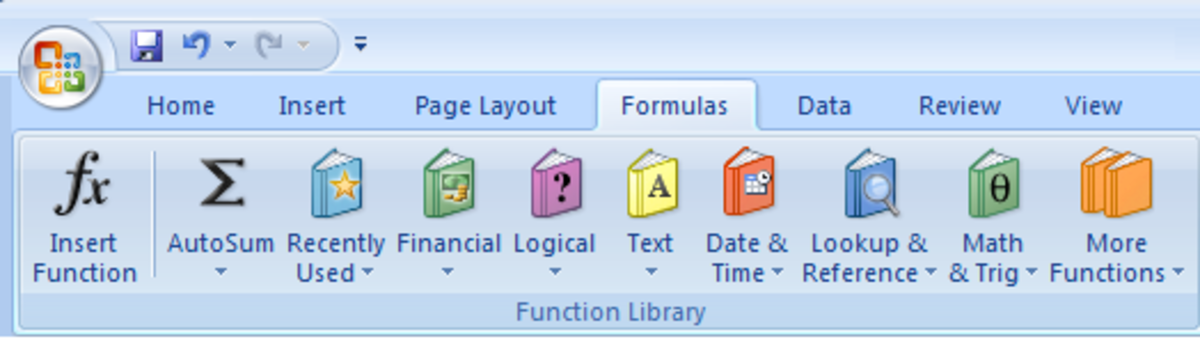The Function Library in Excel 2007 and Excel 2010 logically groups functions together so that they can be easily found.http://robbiecwilson.hubpages.com/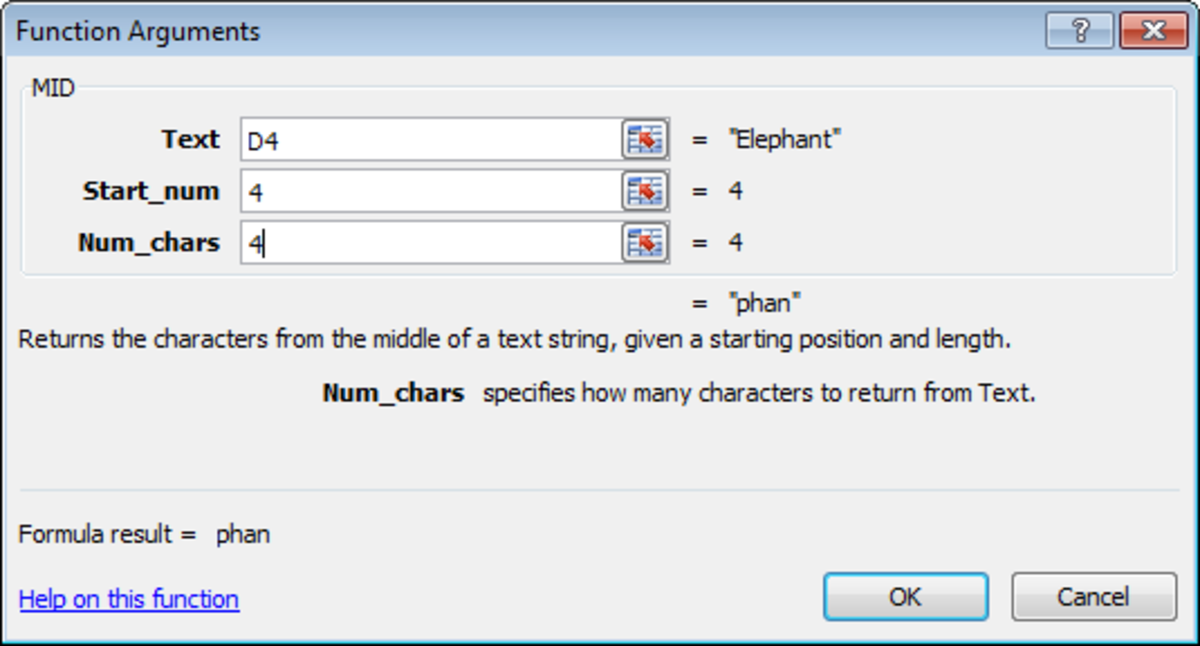The Insert Function tool in Excel 2007 and Excel 2010 which assists you to easily build formulas.http://robbiecwilson.hubpages.com/

## Using the Insert Function to Search for the Best Function for You to Use in Excel 2007 and Excel 2010

The Insert Function tool allows you to search for a function, or to choose one from a drop-down list. In our first example, we have a short list of numbers, and we would like Excel to tell us which of those numbers is the biggest.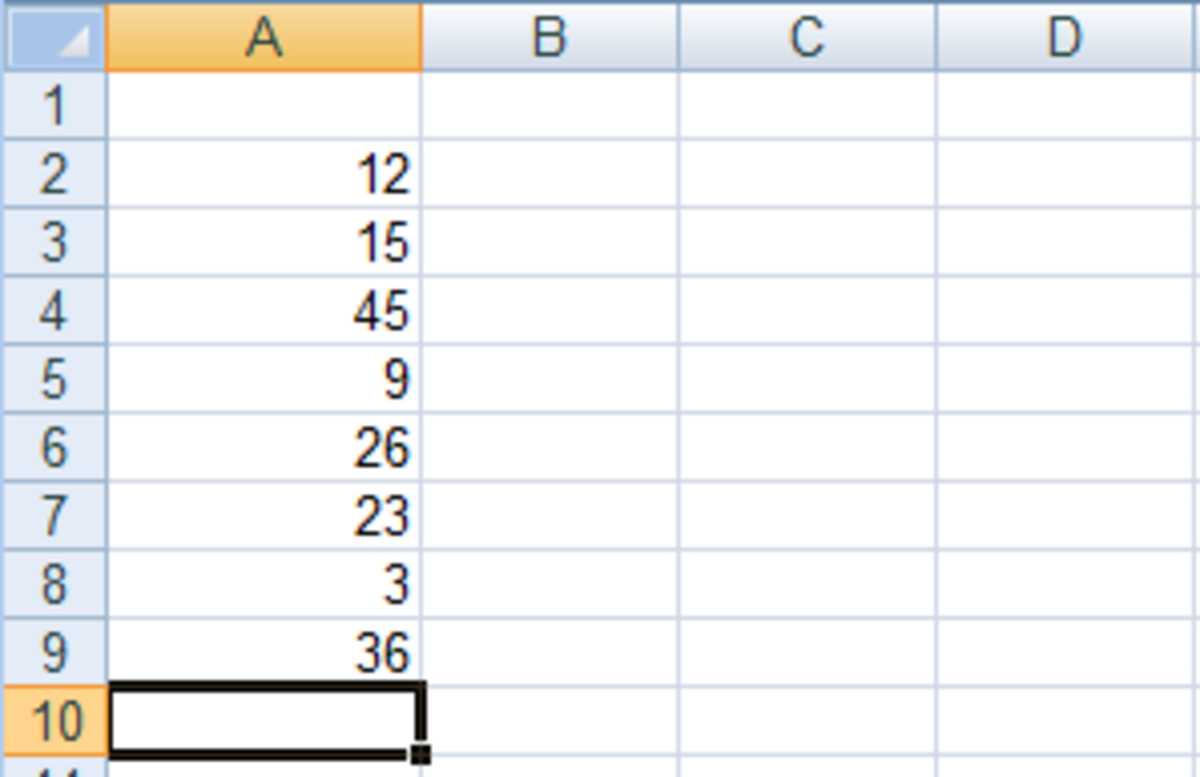Source data from which we will use a the Insert Function tool to find a function to tell us which cell contains the highest number in Excel 2007 or Excel 2010.http://robbiecwilson.hubpages.com/

I select cell A10 and then click the Insert Function button, which is in the Function Library group on the Formulas tab.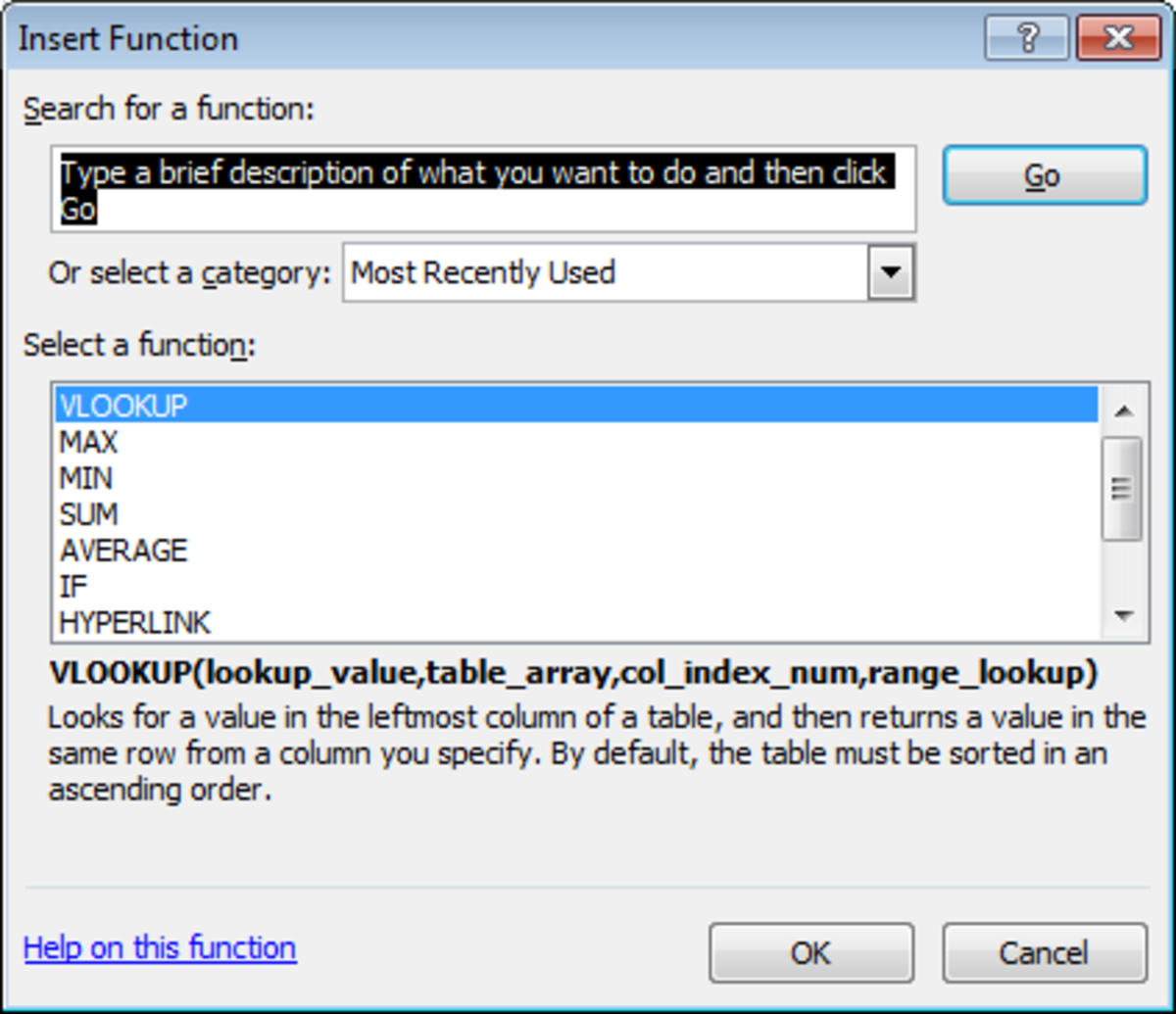Using the Insert Function tool's search capability to find the best function for our formula in Excel 2007 or Excel 2010.http://robbiecwilson.hubpages.com/

We don’t know what formula we want to use, so in the Search for a function box type in Biggest Number.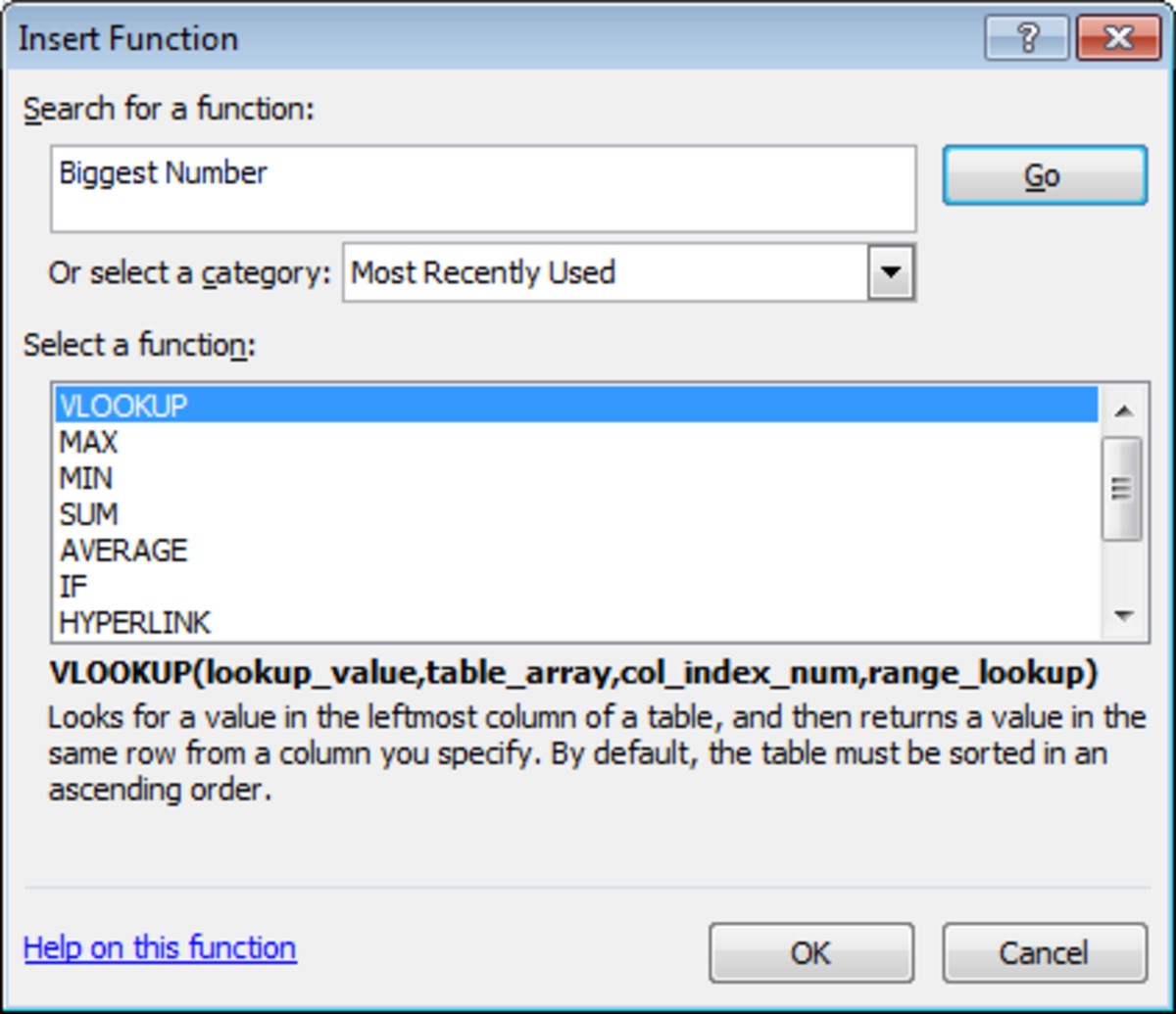Searching for the appropriate function for our formula using search in the Insert function tool in Excel 2007 or Excel 2010.http://robbiecwilson.hubpages.com/
• Click Go

Excel then comes back with some suggestions based on what we asked:

• The first is DMAX which according to the description is used to return the largest number in the column of records in a database. As we don’t have a database, we will keep looking down the list.
• The next function is MAXA. This function returns the largest value but does not ignore logical values and text, so we will skip this too as we want it to ignore any cells containing text.
• MAX is the next in the list. This also returns the largest value and does ignore logical values and text so we will choose MAX.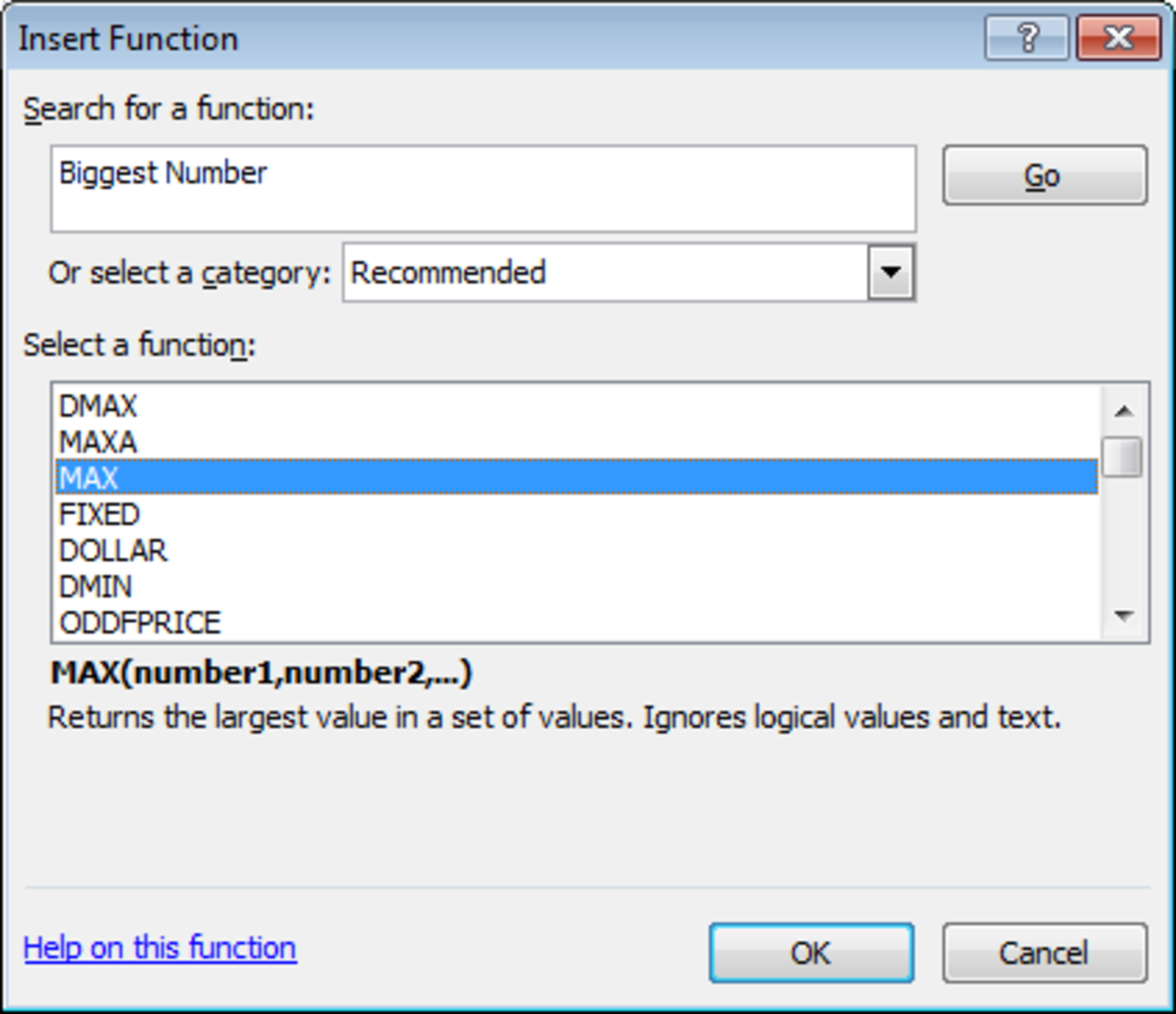The Insert Function tool in Excel 2007 or Excel 2010 has enabled us to quickly and easily find the function that we need .http://robbiecwilson.hubpages.com/
Scroll to Continue
• Select MAX
• Click OK

Excel now shows how clever the Insert Function tool is. Without me even typing anything in, it has completed the formula for me based on the cells nearby the cell containing the formula.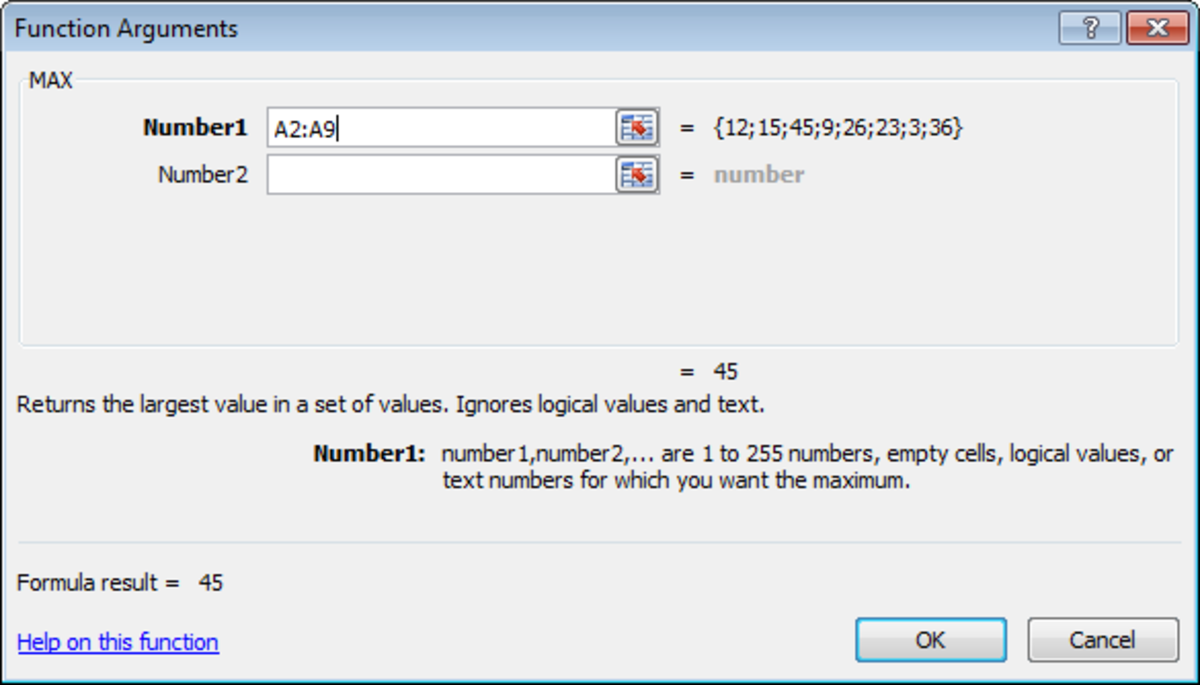The Insert Function has auto-completed our formula in Excel 2007 or Excel 2010 based on the function we chose and the surrounding cells in our spreadsheet.http://robbiecwilson.hubpages.com/

Excel shows you the answer that your current formula will give you as well as explaining once more what the function does.

It also gives you an example of what to put into the Number1 field should you wish to change what Excel has already inputted for you.

If we click OK, you can see that we have created a formula using the MAX function without even typing anything in!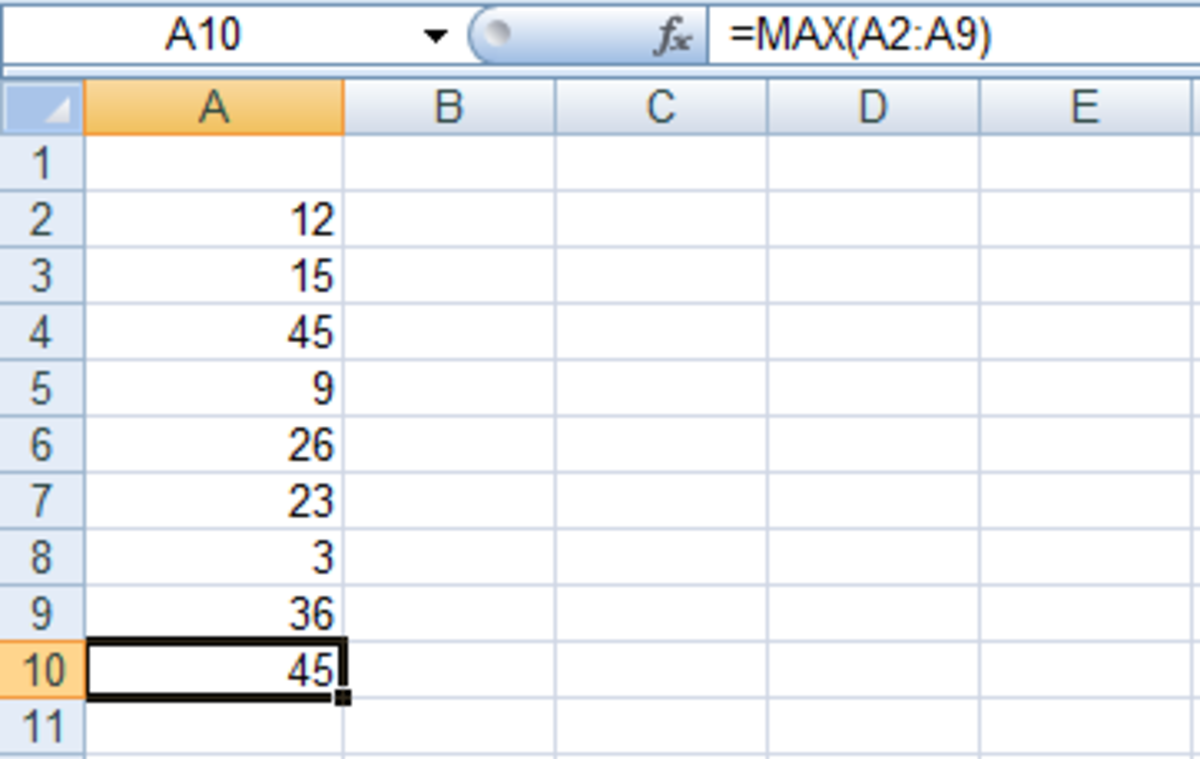Formula created using the Insert Function tool in Excel 2007 or Excel 2010.http://robbiecwilson.hubpages.com/

## Using the Function Library to Create a Formula in Excel 2007 and Excel 2010

Excel collects functions together in the library into a number of groups on the Formulas tab. These include:

• Recently Used – Excel stores functions you have recently used here
• Financial – e.g., NPV (Net Present Value), PV (Present Value)
• Logical – e.g., IF, AND OR
• Text – e.g., CONCATENATE (join together content from different cells) and LEN (find out the number of characters in a cell)
• Date & Time
• Lookup & Reference – e.g., CHOOSE and VLOOKUP
• Math & Trig – e.g., SIN, TAN and DEGREES
• More Functions – Includes Statistical (e.g., AVERAGE, MAX), Engineering, Cube and Informational (e.g., ISBLANK, ISERROR) functions

If you know which group your formula is in, you can open that group and then select the function you would like to use from the list.

Note: If you can’t find the function you are looking for, each group has the Insert Function tool we used above at the bottom of the list.

For this example, we will use the MID function from the Text group.

We have the word Elephant in cell D4, and we want to extract some characters from the middle of it.

• I click on D5 and then select the Text button from the Functional Library group
• Select MID from the drop down list
• Each dialogue box has an explanation of what is expected as we saw above with MAX
• Select Text and then cell D4 which contains the text Elephant
• Start_num is the position of the first character you want to extract; we will choose 4 for this
• Num_chars is the number of characters you want to extract, we will again choose 4

Excel now displays the Formula result (which is phan in this example). If this is not the result we want, we can change any of the above boxes to change the result

• Once you are happy, click OK

You can see once again, Excel has created the formula for us and the results are displayed in cell D5.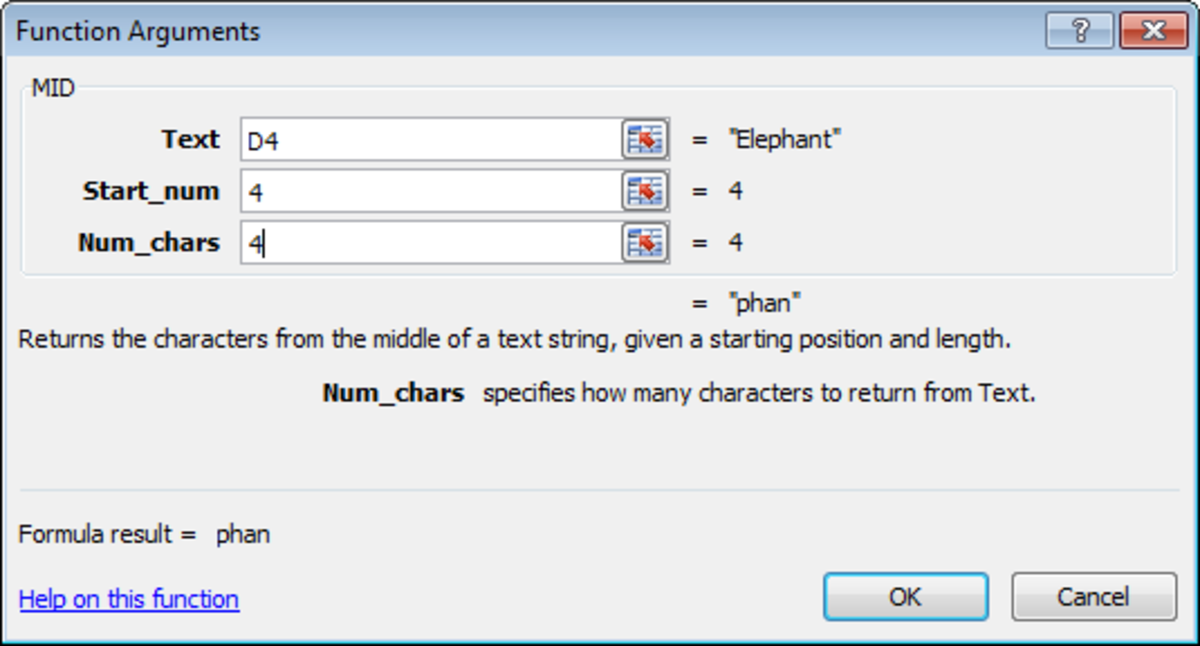Constructing a formula using the MID function using the Function Library in Excel 2007 or Excel 2010. http://robbiecwilson.hubpages.com/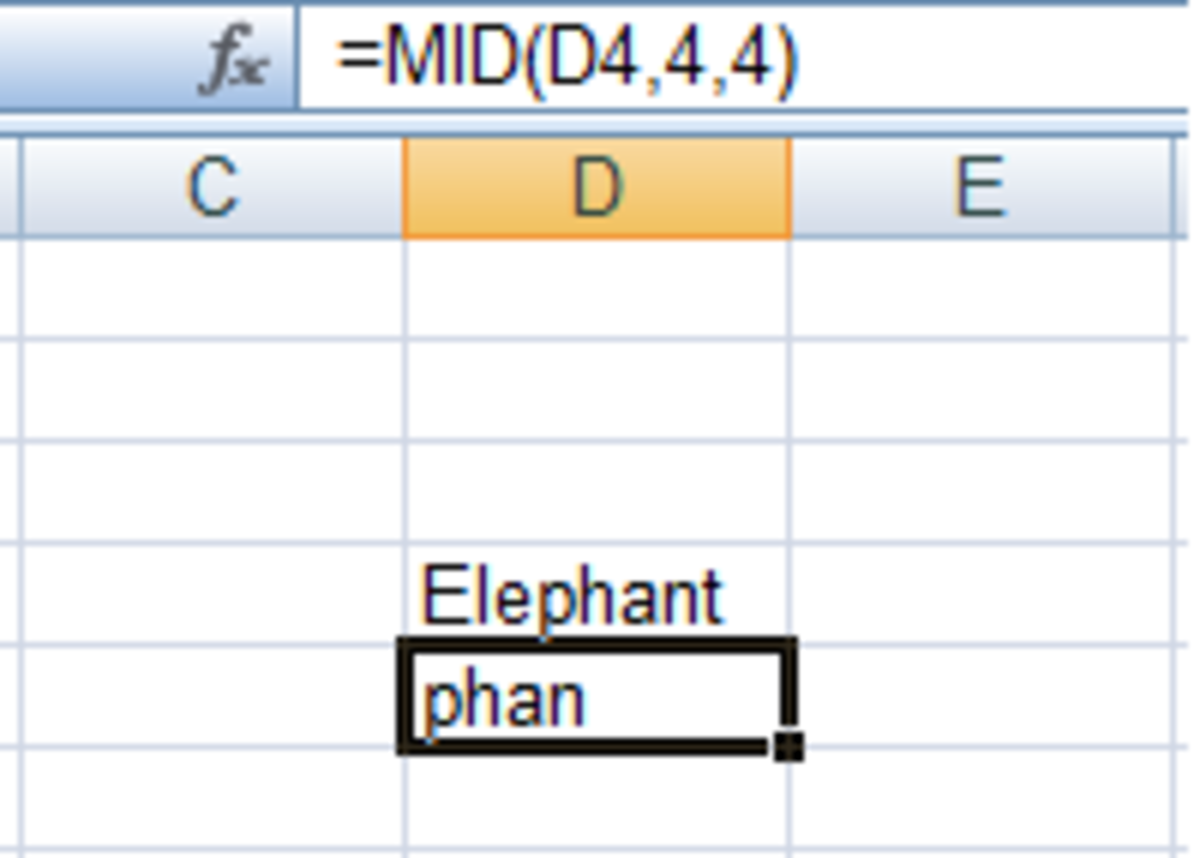A completed formula in Excel 2007 or Excel 2010 created using the Function Library.http://robbiecwilson.hubpages.com/

To assist users in navigating the numerous functions that Excel has available, Microsoft has introduced two tools into Excel.

• First, they have grouped all of their functions into logical groups to create the Function Library.
• Secondly, within the Insert Function tool, you can search for the best function for your situation (we searched for biggest number in our first example)
• Lastly, again with the Insert Function tool, you can create a formula using the function of your choice. Each part of the formula is represented by a dialogue box complete with instructions to further assist you. You can even adjust the formula easily should the answer provided not meet your expectations

Both of these two tools allow you to create formulas simply and quickly. They allow you to harness the power of Excels wide array of functions without worrying about the syntax or their complexity when using them in formulas. Thanks for reading; I hope that you have found this article useful and informative. Please feel free to leave any comments you may have below.